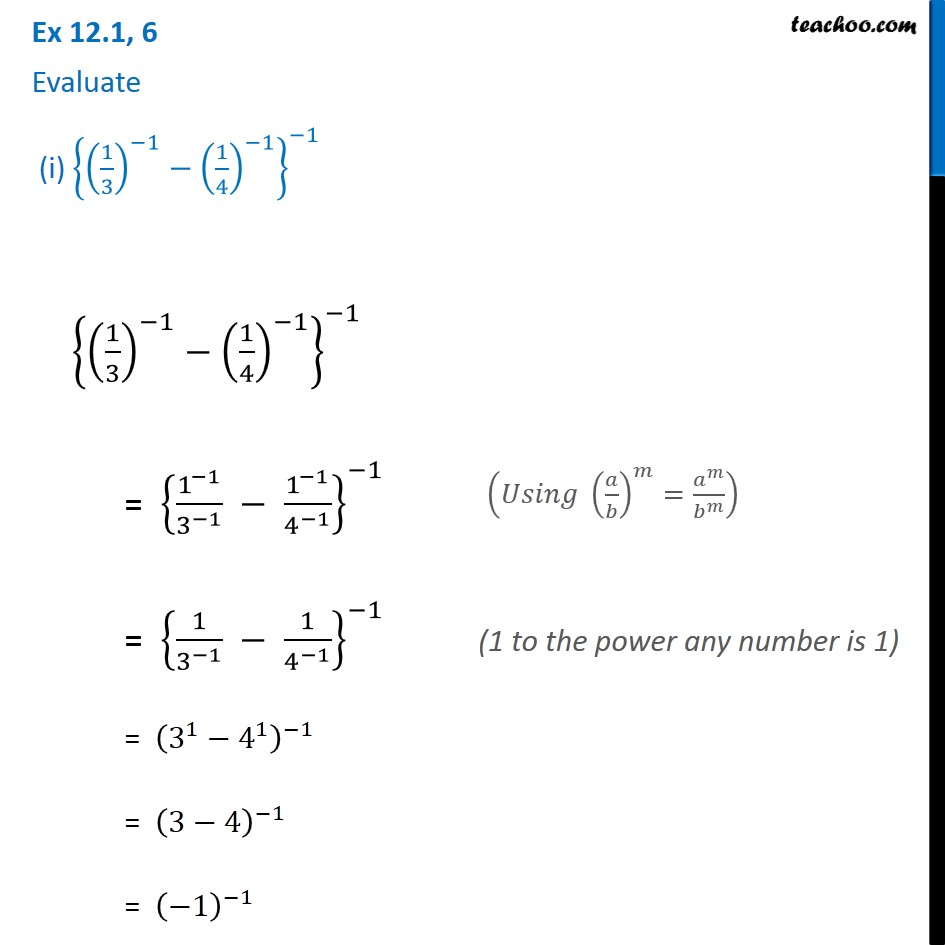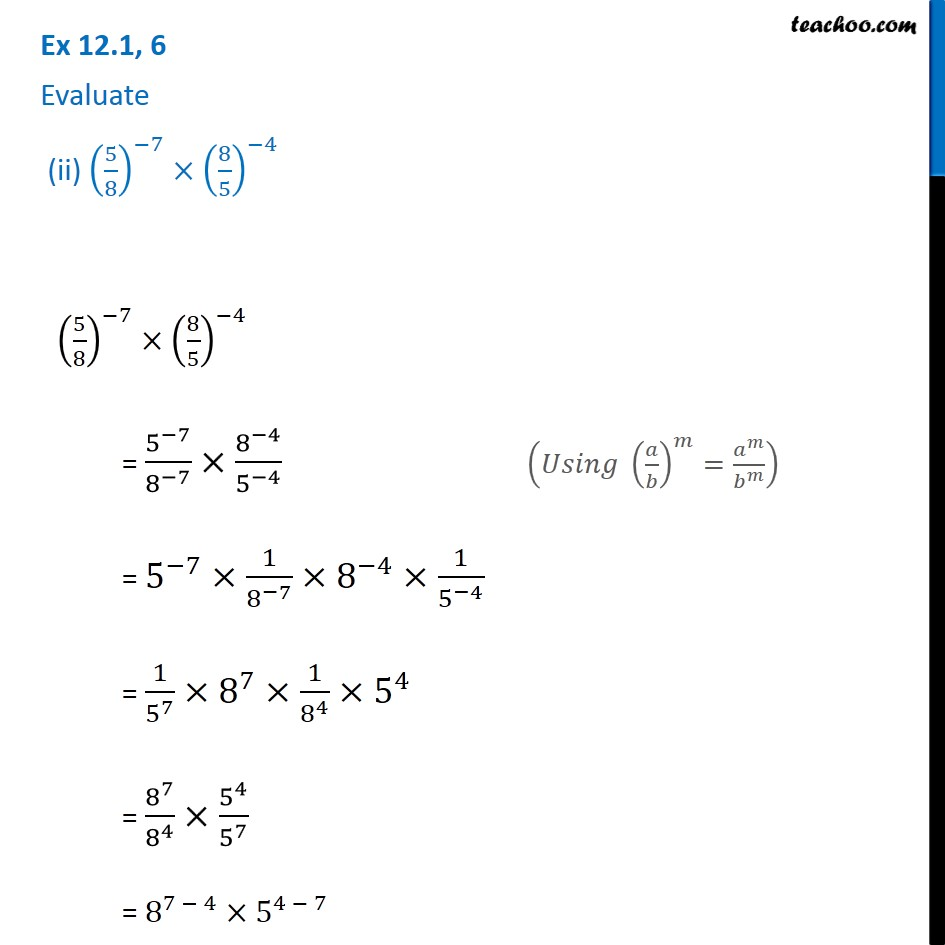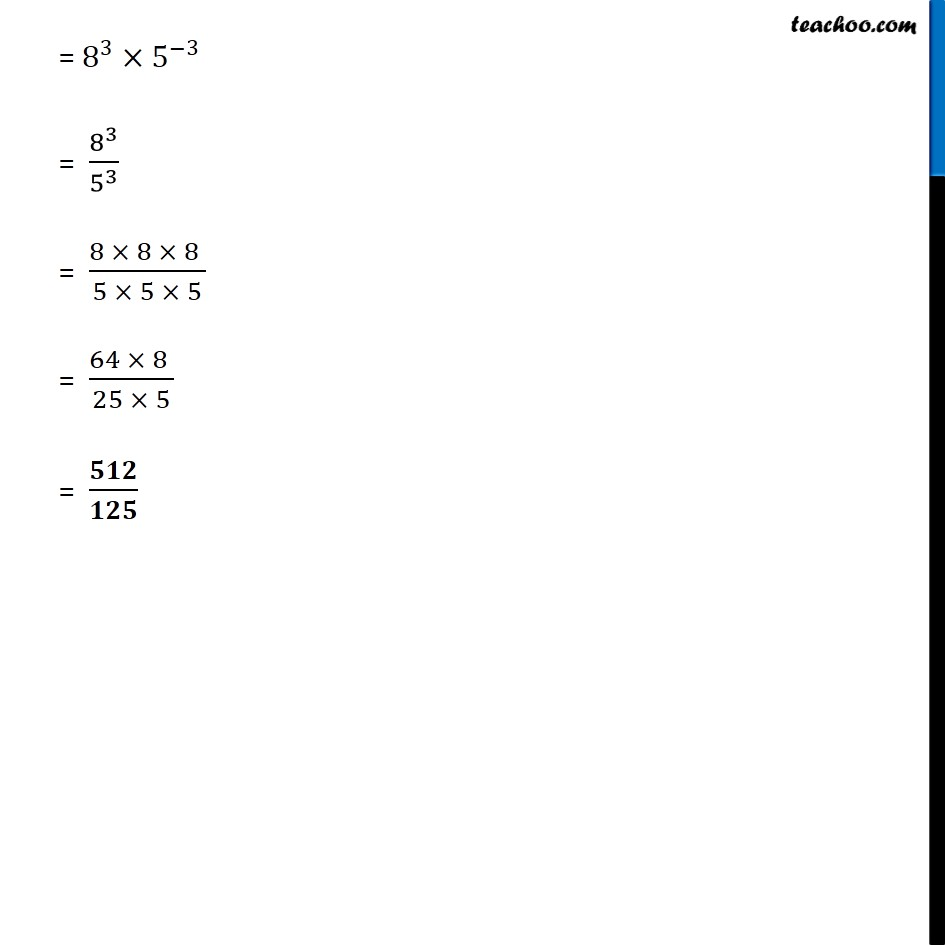Subscribe to our Youtube Channel - https://you.tube/teachoo

1. Chapter 12 Class 8 Exponents and Powers
2. Serial order wise
3. Ex 12.1

Transcript

Ex 12.1, 6 Evaluate (i) {(1/3)^(−1)−(1/4)^(−1) }^(−1) {(1/3)^(−1)−(1/4)^(−1) }^(−1) = {1^(−1)/3^(−1) − 1^(−1)/4^(−1) }^(−1) = {1/3^(−1) − 1/4^(−1) }^(−1) = (3^1−4^1 )^(−1) = (3−4)^(−1) = (−1)^(−1) (𝑈𝑠𝑖𝑛𝑔 (𝑎/𝑏)^𝑚=𝑎^𝑚/𝑏^𝑚 ) (1 to the power any number is 1) = 1/(−1)^1 = 1/(−1) = −𝟏 Ex 12.1, 6 Evaluate (ii) (5/8)^(−7)×(8/5)^(−4) (5/8)^(−7)×(8/5)^(−4) = 5^(−7)/8^(−7) ×8^(−4)/5^(−4) = 5^(−7)×1/8^(−7) ×8^(−4)×1/5^(−4) = 1/5^7 ×8^7×1/8^4 ×5^4 = 8^7/8^4 ×5^4/5^7 = 8^(7 − 4)×5^(4 − 7) (𝑈𝑠𝑖𝑛𝑔 (𝑎/𝑏)^𝑚=𝑎^𝑚/𝑏^𝑚 ) = 8^3×5^(−3) = 8^3/5^3 = (8 × 8 × 8 )/(5 × 5 × 5) = (64 × 8 )/(25 × 5) = 𝟓𝟏𝟐/𝟏𝟐𝟓

Ex 12.1